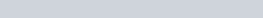You are expected to be able to find the equation of a tangent and normal.

In this video tutorial I introduce you to what a tangent and normal are and show you the general method of finding the respective equations.

This is then followed by the example.

Find the equation of the tangent and normal to:

1.How to find Equations of Tangents and Normals : ExamSolutions Maths Revision - youtube Video

Summary Exercise

Example to try on Equations of Tangents and Normals : ExamSolutions Maths Revision - youtube Video